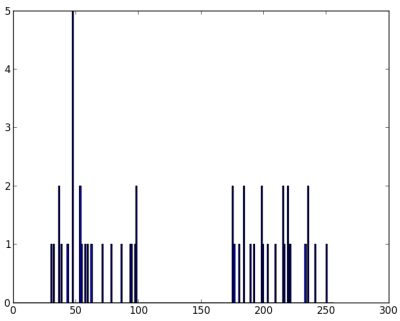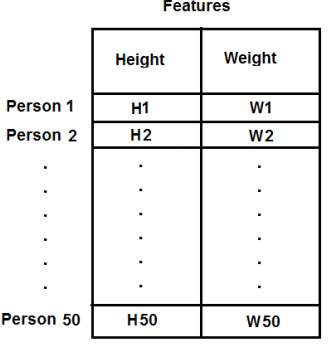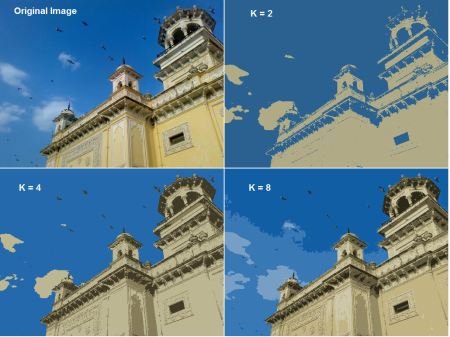OpenCV中的K-Means聚类

### 目标¶

• 了解如何在OpenCV中使用cv.kmeans()函数进行数据聚类

### 理解参数¶

#### 输入参数¶

1. sample：它应该是**np.float32**数据类型，并且每个功能都应该放在单个列中。
2. nclusters(K)：结束条件所需的簇数
3. criteria：这是迭代终止条件。满足此条件后，算法迭代将停止。实际上，它应该是3个参数的元组。它们是(type,max_iter,epsilon)： a. 终止条件的类型。它具有3个标志，如下所示：

• cv.TERM_CRITERIA_EPS-如果达到指定的精度epsilon，则停止算法迭代。
• cv.TERM_CRITERIA_MAX_ITER-在指定的迭代次数max_iter之后停止算法。
• cv.TERM_CRITERIA_EPS + cv.TERM_CRITERIA_MAX_ITER-当满足上述任何条件时，停止迭代。

b. max_iter-一个整数，指定最大迭代次数。 c. epsilon-要求的精度 1. attempts：该标志用于指定使用不同的初始标签执行算法的次数。该算法返回产生最佳紧密度的标签。该紧凑性作为输出返回。 2. flags：此标志用于指定初始中心的获取方式。通常，为此使用两个标志：cv.KMEANS_PP_CENTERS**和**cv.KMEANS_RANDOM_CENTERS

### 1. 单特征数据¶

import numpy as np
import cv2 as cv
from matplotlib import pyplot as plt
x = np.random.randint(25,100,25)
y = np.random.randint(175,255,25)
z = np.hstack((x,y))
z = z.reshape((50,1))
z = np.float32(z)
plt.hist(z,256,[0,256]),plt.show()# 定义终止标准 = ( type, max_iter = 10 , epsilon = 1.0 )
criteria = (cv.TERM_CRITERIA_EPS + cv.TERM_CRITERIA_MAX_ITER, 10, 1.0)
# 设置标志
flags = cv.KMEANS_RANDOM_CENTERS
# 应用K均值
compactness,labels,centers = cv.kmeans(z,2,None,criteria,10,flags)


A = z[labels==0]
B = z[labels==1]


# 现在绘制用红色'A'，用蓝色绘制'B'，用黄色绘制中心
plt.hist(A,256,[0,256],color = 'r')
plt.hist(B,256,[0,256],color = 'b')
plt.hist(centers,32,[0,256],color = 'y')
plt.show()


### 2. 多特征数据¶import numpy as np
import cv2 as cv
from matplotlib import pyplot as plt
X = np.random.randint(25,50,(25,2))
Y = np.random.randint(60,85,(25,2))
Z = np.vstack((X,Y))
# 将数据转换未 np.float32
Z = np.float32(Z)
# 定义停止标准，应用K均值
criteria = (cv.TERM_CRITERIA_EPS + cv.TERM_CRITERIA_MAX_ITER, 10, 1.0)
ret,label,center=cv.kmeans(Z,2,None,criteria,10,cv.KMEANS_RANDOM_CENTERS)
# 现在分离数据, Note the flatten()
A = Z[label.ravel()==0]
B = Z[label.ravel()==1]
# 绘制数据
plt.scatter(A[:,0],A[:,1])
plt.scatter(B[:,0],B[:,1],c = 'r')
plt.scatter(center[:,0],center[:,1],s = 80,c = 'y', marker = 's')
plt.xlabel('Height'),plt.ylabel('Weight')
plt.show()


### 3.颜色量化¶

import numpy as np
import cv2 as cv
Z = img.reshape((-1,3))
# 将数据转化为np.float32
Z = np.float32(Z)
# 定义终止标准 聚类数并应用k均值
criteria = (cv.TERM_CRITERIA_EPS + cv.TERM_CRITERIA_MAX_ITER, 10, 1.0)
K = 8
ret,label,center=cv.kmeans(Z,K,None,criteria,10,cv.KMEANS_RANDOM_CENTERS)
# 现在将数据转化为uint8, 并绘制原图像
center = np.uint8(center)
res = center[label.flatten()]
res2 = res.reshape((img.shape))
cv.imshow('res2',res2)
cv.waitKey(0)
cv.destroyAllWindows()